Documentation

# settlingtime

Settling time for bilevel waveform

## Syntax

```S = settlingtime(X,D) S = settlingtime(X,FS,D) S = settlingtime(X,T,D) [S,SLEV,SINST] = settlingtime(...) [S,SLEV,SINST] = settlingtime(...,Name,Value) settlingtime(...) ```

## Description

`S = settlingtime(X,D)` returns the time, `S`, from the mid-reference level instant to the time instant each transition enters and remains within a 2% tolerance region of the final state over the duration, `D`. `D` is a positive scalar. Because `settlingtime` uses interpolation to determine the mid-reference level instant, `S` may contain values that do not correspond to sampling instants. The length of `S` is equal to the number of detected transitions in the input signal, `X`. If for any transition, the level of the waveform does not remain within the lower and upper tolerance boundaries, the requested duration is not present, or an intervening transition is detected, `settlingtime` marks the corresponding element in `S` as `NaN`. See Settle Seek Duration for cases in which `settlingtime` returns a `NaN`. To determine the transitions, `settlingtime` estimates the state levels of the input waveform by a histogram method. `settlingtime` identifies all regions that cross the upper-state boundary of the low state and the lower-state boundary of the high state. The low-state and high-state boundaries are expressed as the state level plus or minus a multiple of the difference between the state levels. See State-Level Tolerances.

`S = settlingtime(X,FS,D)` specifies the sample rate for the bilevel waveform, `X` in hertz. The first sample instant in `X` is equal to t = 0. Because `settlingtime` uses interpolation to determine the mid-reference level instant, `S` may contain values that do not correspond to sampling instants.

`S = settlingtime(X,T,D)` specifies the sample instants, `T`, as a vector with the same number of elements as `X`.

``` [S,SLEV,SINST] = settlingtime(...)``` returns vectors, `SLEV`, and `SINST`, whose elements correspond to the levels and sample instants of the settling points for each transition.

``` [S,SLEV,SINST] = settlingtime(...,Name,Value)``` returns the settling times, levels, and corresponding sample instants with additional options specified by one or more `Name,Value` pair arguments.

`settlingtime(...)` plots the signal and darkens the regions of each transition where settling time is computed. The plot marks the location of the settling time of each transition, the mid-crossings, and the associated reference levels. The plot also displays the state levels with the corresponding lower and upper tolerance boundaries.

## Input Arguments

 `X` Bilevel waveform. `X` is a real-valued row or column vector. `D` Settle-seek duration. `D` is a positive scalar, which defines the duration after the mid-reference level instant that `settlingtime` looks for a settling time. If no settling time occurs in `D` seconds after the mid-reference level instant, `settlingtime` returns a `NaN`. See Settling Time and Settle Seek Duration. `FS` Sample rate in hertz. `T` Vector of sample instants. The length of `T` must equal the length of the bilevel waveform, `X`.

### Name-Value Pair Arguments

 `'MidPercentReferenceLevel'` Mid-reference level as a percentage of the waveform amplitude. See Mid-Reference Level. Default: `50` `'StateLevels'` Low and high-state levels. `StateLevels` is a 1-by-2 real-valued vector. The first element is the low-state level. The second element is the high-state level. If you do not specify low and high-state levels, `settlingtime` estimates the state levels from the input waveform using the histogram method. `'Tolerance'` Tolerance levels (lower and upper state boundaries) expressed as a percentage. See State-Level Tolerances. Default: `2`

## Output Arguments

 `S` The time from the mid-reference level instant to the time instant each transition enters and remains within a 2% tolerance region of the final state over duration, `D`. `SLEV` Waveform values at the settling points. `SINST` Time instants of the settling points.

## Examples

collapse all

Determine the settling point and corresponding waveform value for a bilevel waveform. Specify a settle-seek duration of 10 seconds.

```load('transitionex.mat', 'x') [s,slev,sinst] = settlingtime(x,10);```

Plot the waveform and annotate the settling point.

`settlingtime(x,10)`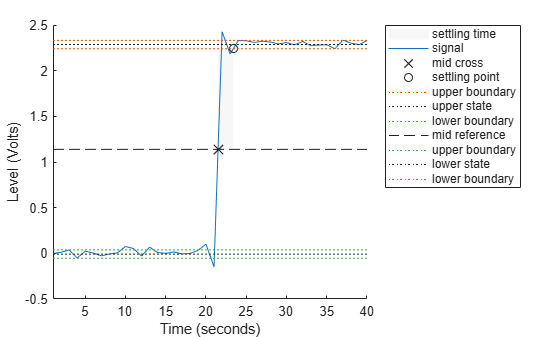```ans = 1.8901 ```

Determine the settling points for a three-transition bilevel waveform. The data are sampled at 4 MHz. Specify a settle-seek duration of one microsecond.

```load('transitionex.mat','x') y = [x; fliplr(x)]; fs = 4e6; t = 0:1/fs:(length(y)*1/fs)-1/fs; [s,slev,sinst] = settlingtime(y,fs,1e-6);```

Plot the waveform and annotate the settling points.

`settlingtime(y,fs,1e-6)`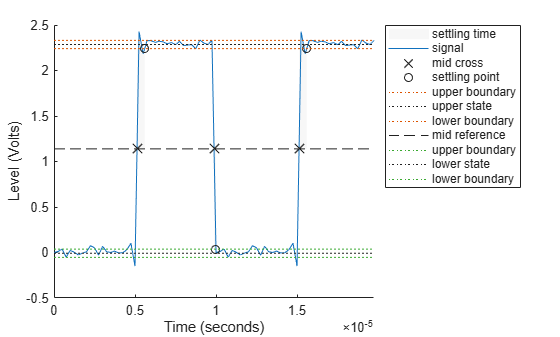```ans = 3×1 10-6 × 0.4725 0.1181 0.4725 ```

collapse all

### Settling Time

The settling time is the time after the mid-reference level instant when the signal crosses into and remains in the 2%-tolerance region around the state level. The settling time is illustrated in the following figure. The low- and high-state levels are the dashed black lines. The 2% tolerances above and below the state levels are shown by the red dashed lines and the settling time is indicated by the yellow circle.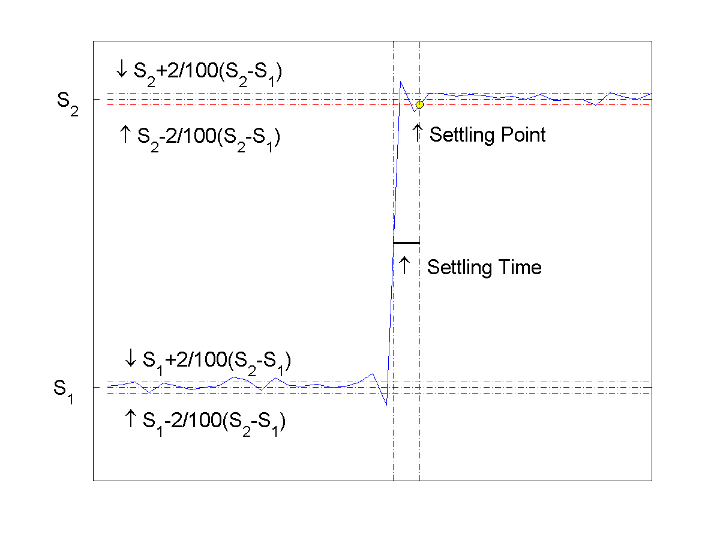### Mid-Reference Level

The mid-reference level in a bilevel waveform with low-state level, S_1, and high- state level, S_2, is

`${S}_{1}+\frac{1}{2}\left({S}_{2}-{S}_{1}\right)$`

### Mid-Reference Level Instant

Let y50% denote the mid reference level.

Let t50%- and t50%+ denote the two consecutive sampling instants corresponding to the waveform values nearest in value to y50%.

Let y50%- and y50%+ denote the waveform values at t50%- and t50%+.

The mid-reference level instant is

`${t}_{50%}={t}_{50%}+\left(\frac{{t}_{50{%}_{+}}-{t}_{50{%}_{-}}}{{y}_{50{%}_{+}}-{y}_{50{%}_{-}}}\right)\left({y}_{50{%}_{+}}-{y}_{50{%}_{-}}\right)$`

### State-Level Tolerances

Each state level can have associated lower- and upper-state boundaries. These state boundaries are defined as the state level plus or minus a scalar multiple of the difference between the high state and the low state. To provide a useful tolerance region, the scalar is typically a small number such as 2/100 or 3/100. In general, theregion for the low state is defined as

``

whereis the low-state level andis the high-state level. Replace the first term in the equation withto obtain thetolerance region for the high state.

The following figure illustrates lower and upper 2% state boundaries (tolerance regions) for a positive-polarity bilevel waveform. The red dashed lines indicate the estimated state levels.### Settle Seek Duration

The settle seek duration defines the interval of time after the mid-reference level instant that `settlingtime` looks for a settling point. If `settlingtime` does not find a settling point within the settle seek duration, `settlingtime` returns `NaN` for the settling time. The following figure illustrates a settle seek duration of 10 samples.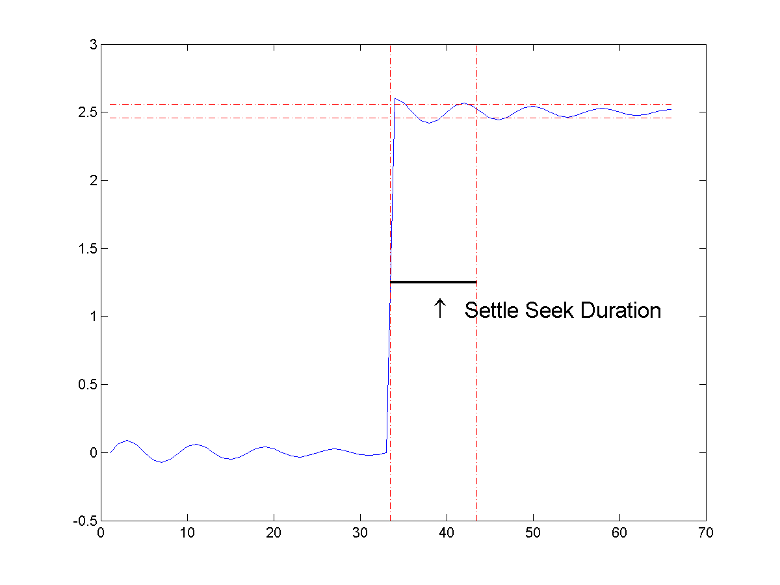`settlingtime` may fail to find a settling point in the specified settle seek duration if any one of the following conditions occurs:

• The last waveform value in the settle seek interval is not within the upper- and lower-state boundaries determined by the specified tolerance. The following figure illustrates this condition for a settle seek duration of 8 samples and a 2% tolerance region.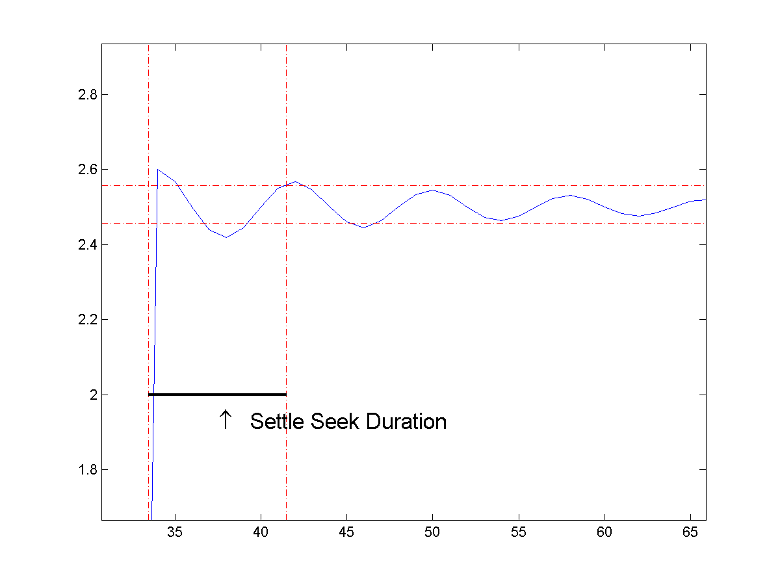In the preceding figure, you see that the last sample in the settle seek interval exceeds the upper state boundary. In this example, reducing or increasing the settle seek duration can result in a valid settling time.

• There is an insufficient number of waveform samples for the specified settle seek duration. The following figure illustrates this condition for a settle seek duration of 20 samples. The settle seek duration extends beyond the final sample of the waveform.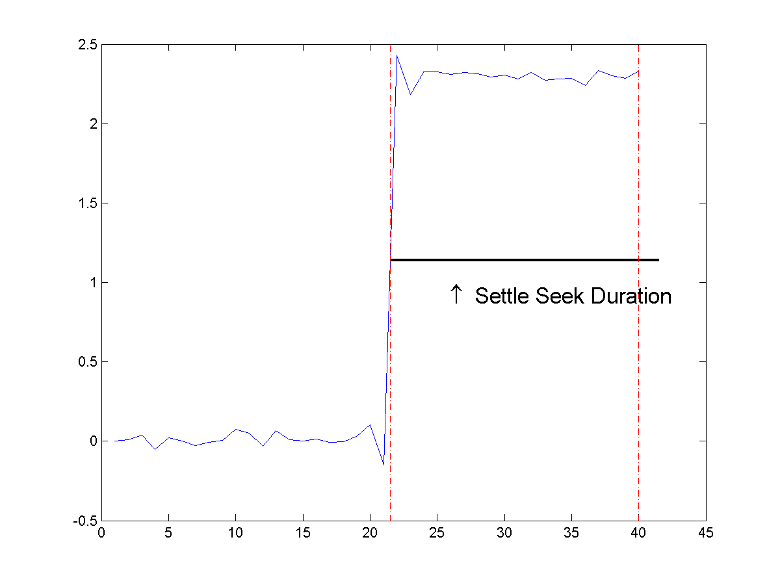• An intervening transition is detected before the end of the specified settle seek duration. The following figure illustrates this condition for a settle seek duration of 22 samples. An intervening transition is detected before the end of the 22–sample settle seek duration.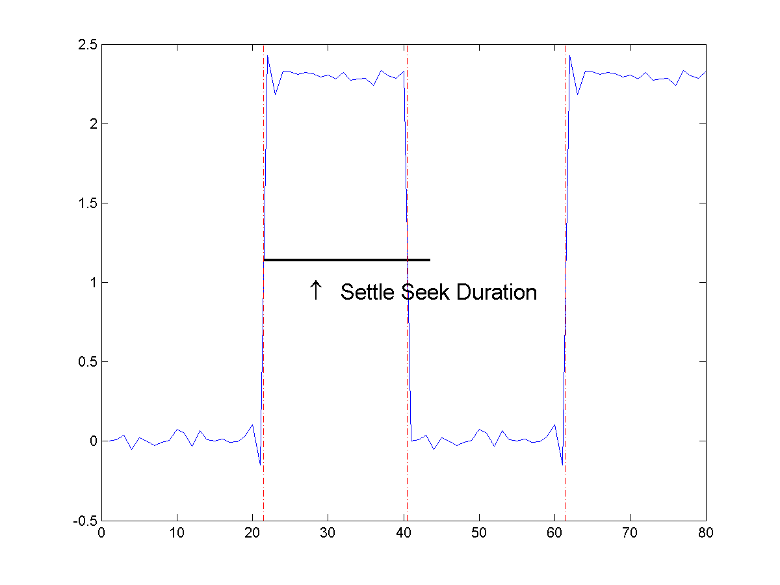## References

 IEEE® Standard on Transitions, Pulses, and Related Waveforms, IEEE Standard 181, 2003, pp. 23–24.